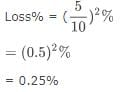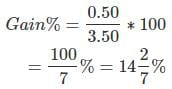Test: Profit & Loss- 2

# Test: Profit & Loss- 2

Test Description

## 10 Questions MCQ Test CSAT Preparation for UPSC CSE | Test: Profit & Loss- 2

Test: Profit & Loss- 2 for Banking Exams 2022 is part of CSAT Preparation for UPSC CSE preparation. The Test: Profit & Loss- 2 questions and answers have been prepared according to the Banking Exams exam syllabus.The Test: Profit & Loss- 2 MCQs are made for Banking Exams 2022 Exam. Find important definitions, questions, notes, meanings, examples, exercises, MCQs and online tests for Test: Profit & Loss- 2 below.
Solutions of Test: Profit & Loss- 2 questions in English are available as part of our CSAT Preparation for UPSC CSE for Banking Exams & Test: Profit & Loss- 2 solutions in Hindi for CSAT Preparation for UPSC CSE course. Download more important topics, notes, lectures and mock test series for Banking Exams Exam by signing up for free. Attempt Test: Profit & Loss- 2 | 10 questions in 20 minutes | Mock test for Banking Exams preparation | Free important questions MCQ to study CSAT Preparation for UPSC CSE for Banking Exams Exam | Download free PDF with solutions
 1 Crore+ students have signed up on EduRev. Have you?
Test: Profit & Loss- 2 - Question 1

### A man sells two houses at the rate of Rs.1.995 lakhs each. On one he gains 5% and on the other, he loses 5%. What is his gain or loss percent in the whole transaction?

Detailed Solution for Test: Profit & Loss- 2 - Question 1

The correct answer is A asTest: Profit & Loss- 2 - Question 2

### A trader gives 12% additional discount on the discounted price, after giving an initial discount of 20% on the labeled price of an item. The final sale price of the item is Rs.704. Find out the labeled price?

Detailed Solution for Test: Profit & Loss- 2 - Question 2

Let the marked price be Rs. 100.
Successive discounts of 20% and 12% were given.
Sale price = 100 × 80/100 × 88/100 = Rs.704/10
If the sale price is Rs.704/10, the marked price is Rs.100
If the sale price is Rs.704, the marked price is
= 100 × 10 × 704/704 =  Rs.1000

Test: Profit & Loss- 2 - Question 3

### A trader mixes 26 kg of rice at Rs. 20 per kg with 30 kg of rice of other variety at Rs. 36 per kg and sells the mixture at Rs. 30 per kg. What is his profit percentage?

Detailed Solution for Test: Profit & Loss- 2 - Question 3

C.P. of 56 kg rice = Rs. (26 x 20 + 30 x 36) = Rs. (520 + 1080) = Rs. 1600.

S.P. of 56 kg rice = Rs. (56 x 30) = Rs. 1680.

Gain =(80/1600*100) % = 5%

Test: Profit & Loss- 2 - Question 4

On selling 17 balls at Rs. 720, there is a loss equal to the cost price of 5 balls. What is the cost price of a ball?

Detailed Solution for Test: Profit & Loss- 2 - Question 4

Let the cost price of a ball be Rs. x.

So, the CP of 17 balls is Rs. 17x.

The CP of 5 balls is Rs. 5x.

Since Loss = CP - SP

So, 5x = 17x - 720

On solving, we get

12 x = 720

that is, x = 60

So, the cost price of a ball is Rs. 60.

Test: Profit & Loss- 2 - Question 5

If a material is sold for Rs.34.80, there is a loss of 25%. Find out the cost price of the material?

Detailed Solution for Test: Profit & Loss- 2 - Question 5

The correct answer is A as
cost price
=34.80×100/75
=46.40

Test: Profit & Loss- 2 - Question 6

A shopkeeper sells one more radio for Rs. 840 at a gain of 20% and another for Rs. 960 at a loss of 4%. What is his total gain or loss percentage?

Detailed Solution for Test: Profit & Loss- 2 - Question 6

Case 1) S.P of transistor = Rs 840
Profit = 20%
By unitary method C.P = (100/120) ×840= 700Rs
Case 2) S.P of transistor = Rs960
Loss = 4%
By unitary method C.P = (100/96) × 960 = 1000 Rs
Total C.P = 700 + 1000 =1700 Rs
Total S.P= 840 +960 = 1800Rs
There is a profit of = 1800 - 1700 = Rs100
Therefore profit% = (100/1700) × 100 = 100/17
= 5.882% Ans

Test: Profit & Loss- 2 - Question 7

John purchased a machine for Rs. 80,000. After spending Rs.5000 on repair and Rs.1000 on transport he sold it with 25% profit. What price did he sell the machine?

Detailed Solution for Test: Profit & Loss- 2 - Question 7

C.P. = (80000 + 5000 + 1000)
⇒ 86000
As we know that Profit = 25%
S.P. = 125% of 86000
⇒ (125/100 * 86000)
⇒ 107500 Rs.

Test: Profit & Loss- 2 - Question 8

100 oranges were brought at the rate of Rs. 350 and sold at the rate of Rs. 48 per dozen. What is the percentage of profit or loss?

Detailed Solution for Test: Profit & Loss- 2 - Question 8

So before solving this question we will get the C.P. and S.P. of 1 article to get the gain percent.

C.P. of 1 orange = 350/100 = Rs 3.50
S.P. of one orange = 48/12 = Rs 4 [note: divided by 12 as 1 dozen contains 12 items]
Gain = 4 - 3.50 = Rs 0.50Profit

Test: Profit & Loss- 2 - Question 9

When an item is sold for Rs. 18,700, the owner loses 15%. At what price should that plot be sold to get a gain of 15%?

Detailed Solution for Test: Profit & Loss- 2 - Question 9

85 : 18700 = 115 : x
⇒ x = (18700*115)/85
x = 25300

Test: Profit & Loss- 2 - Question 10

A fruit seller sells apples at the rate of Rs.9 per kg and thereby loses 20%. At what price per kg, he should have sold them to make a profit of 5%?

## CSAT Preparation for UPSC CSE

72 videos|64 docs|92 tests
 Use Code STAYHOME200 and get INR 200 additional OFF Use Coupon Code
Information about Test: Profit & Loss- 2 Page
In this test you can find the Exam questions for Test: Profit & Loss- 2 solved & explained in the simplest way possible. Besides giving Questions and answers for Test: Profit & Loss- 2, EduRev gives you an ample number of Online tests for practice

## CSAT Preparation for UPSC CSE

72 videos|64 docs|92 tests